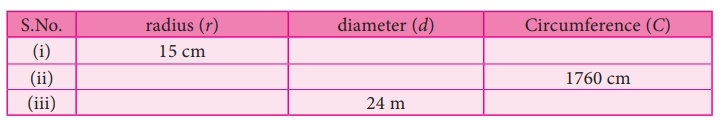Home | | Maths 7th Std | Exercise 2.1 (Circumference of a Circle)

# Exercise 2.1 (Circumference of a Circle)

7th Maths : Term 2 Unit 2 : Measurements : Circumference of a Circle : Exercise 2.1 : Text Book Back Exercises Questions with Answers, Solution

Exercise 2.1

1. Find the missing values in the following table for the circles with radius (r), diameter (d) and Circumference (C).i) Radius r = 15 cm

Diameter d = 2r = 2 × l5 = 30cm

Circumference C = 2πr

= 2 × 22/7 × 15 cm = 660/7cm

= 94.28 cm

ii) Circumference C = 1760 cm

2πr = 1760

2 × 22/7 × r = 1760

r = 1760×7 / 2×22 = 280 cm

Diameter d = 2r = 2 × 280 = 560 cm

iii) Diameter d = 24 m

radius r = d/2 = 24/2 = 12 m

Circumference C= 2πr

= 2 × 22/7 × 12 cm

= 528 / 7 = 75.42 m2. Diameters of different circles are given below. Find their circumference (Take π = 22/7).

(i) d = 70 cm

Diameter d = 70 cm

Circumference C= πd

= 22/7 × 70 = 220 cm

Circumference = 220 cm

(ii) d = 56 m

Diameter d= 56 cm

Circumference C = πd

= 22/7 × 56 m = 176 m

Circumference = 176 m

(iii) d = 28 mm

Diameter d = 28 mm

Circumference C= πd

= 22/7 × 28 mm = 88 mm

Circumference = 88mm

3. Find the circumference of the circles whose radii are given below.

(i) 49 cm

Circumference C = 2πr

= 2 × 22/7 × 49 = 308 cm

Circumference = 308 cm

(ii) 91 mm

Circumference C = 2πr

= 2 × 22/7 × 91 mm = 572 mm

Circumference = 572 mm

4. The diameter of a circular well is 4.2 m. What is its circumference?

The diameter of a circular well d = 4.2 m

Radius r = d/2 = 42/2 = 2.1m

Circumference C = 2πr

= 2 × 22/7 × 2.1 =13.2 m

Circumference of a circular well = 13.2 m

5. The diameter of the bullock cart wheel is 1.4 m. Find the distance covered by it in 150 rotations?

The diameter of the bullock cart d = 1.4 m

radius r = d/2 = 14/2 = 0.7m

Circumference C = 2πr

= 2 × 22/7 × 0.7m = 4.4m

The distance covered in 1 rotation = 4.4 m

The distance covered in 150 rotation = 4.4 m × 150 = 660m

The distance covered in 150 rotation = 660 m

6. A ground is in the form of a circle whose diameter is 350 m. An athlete makes 4 revolutions. Find the distance covered by the athlete.

Diameter of the ground d = 350 m

radius r = d/2 = 350/2 = 175 m

Circumference C = 2πr

= 2 × 22/7 × 175 m = 1100 m

Distance covered in 1 revolution = 1100 m

Distance covered in 4 revolution = 1100 × 4m = 4400 m

The distance covered by the athlete = 4400 m.

7. A wire of length 1320 cm is made into circular frames of radius 7 cm each. How many frames can be made?

The radius ofthe circular frame r = 7cm

Circumference C = 2πr

= 2 × 22/7 × 7 cm = 44 cm

The Length of the wire = 1320 cm

The Circumference of the frame = 44 cm

Number of Circular frames made = Length of the wire / Circumference of the frame

= 1320 / 44 = 30.

Number of Circular frames made = 30.

8. A Rose garden is in the form of circle of radius 63 m. The gardener wants to fence it at the rate of ₹150 per metre. Find the cost of fencing?

Radius of the rose garden r = 63 m

Circumference C = 2πr

= 2 × 22/7 × 63 m

Circumference of the rose garden = 396 m

The cost of fencing 1 metre = ₹ 150

The cost of fencing 396 metre = 396 × ₹ 150

The cost of fencing = ₹ 59,400

Objective type questions

9. Formula used to find the circumference of a circle is

(i) 2 πr units

(ii) πr2 + 2r units

(iii) πr2 sq. units

(iv) πr3 cu. units

10. In the formula, C = 2πr , ‘r’ refers to

(i) circumference

(ii) area

(iii) rotation

11. If the circumference of a circle is 82π, then the value of ‘r’ is

(i) 41 cm

(ii) 82 cm

(iii) 21 cm

(iv) 20 cm

12. Circumfernce of a circle is always

(i) three times its diameter

(ii) more than three times of its diameter

(iii) less than three times of its diameter

(iv) three times of its radius

Answer : (i) three times of its diameter

Exercise 2.1

1. (i) d = 30 cm; c = 94.28 cm (ii) r = 280 cm; d = 560 cm (iii) r = 12 m; c = 75.42 m

2. (i) 220 cm (ii) 176 m (iii) 88 m

3. (i) 308 cm (ii) 572 mm

4. 13.2 m

5. 660 m

6. 4400 m

7. 30 frames

8. ₹59,400

Objective type questions

9. (i) 2πr units